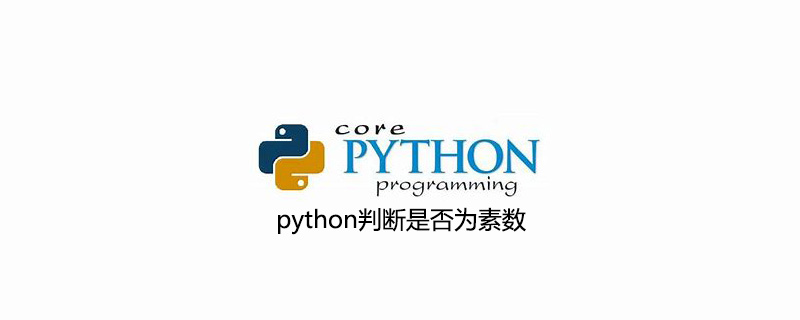• python调用函数判断素数
万次阅读
2021-10-19 17:38:26

### 题目

用函数判断一个数是否是素数

prime(p), 其中函数prime当用户传入参数p为素数时返回True，否则返回False。

### 思路

1、先输入一个要判断的数

2、编写函数，判断素数，输出flag

### 代码

import math
p=eval(input("请输入您要判读是否是素数的数值："))
k=int(math.sqrt(p))
def prime(p):
i=2
flag=True
while (i<=k and flag==True):
if p%i==0:
flag=False
else:
i+=1
return flag
print(prime(p))

### 结果

请输入您要判读是否是素数的数值：6
Falsepython
更多相关内容
• 调用函数，对输入的整数进行判断，如果是素数则输出为True，不是素数则输出为False。 本关涉及的代码文件src/Step2/prime.py的代码框架如下： #coding=utf-8 # 输入一个整数n n = int(input()) # 请在此添加代码，...

### 数学运算

abs()：返回数值的绝对值；
例如：

abs(-4)
4

divmod()：返回两个数值的商和余数；
例如：

divmod(7,2)
(3,1)

max()：返回元素中的最大值；
例如：

max(2,6,1,7)
7

min()：返回元素中的最小值；
例如：

min(2,6,1,7)
1

sum()：返回传入元素之和。
例如：

sum((1,2,3,4))
10
sum([1,2,3,4])
10
sum((1,2,3,4),-10)
0

### 类型转换

bool()：根据传入的参数的逻辑值创建一个新的布尔值；
例如：

bool()
False
bool(1)
True
bool(0)
False
bool(‘str’)
True

int()：根据传入的参数创建一个新的整数；
例如：

int(‘3’)
3
int(‘3.6’)
3

float()：根据传入的参数创建一个新的浮点数；
例如：

float() #不提供参数的时候，返回0.0
0.0
float(3)
3.0
float(‘3’)
3.0

complex()：根据传入的参数创建一个新的复数。
例如：

complex() #当两个参数都不提供时，返回复数0j
0j
complex(‘2+4j’)
(2+4j)
complex(1,2)
(1+2j)

### 序列操作

all()：判断可迭代对象的每个元素是否都为True值；
例如：

all([1,2,3]) #列表中每个元素逻辑值均为True，返回True
True
all([0,1,2]) #列表中0的逻辑值为False，返回False
False
all(()) #空元组
True

any()：判断可迭代对象的元素是否有为True值的元素；
例如：

any([0,1,2]) #列表元素有一个为True，则返回True
True
any([0,0]) #列表元素全部为False，则返回False
False
any([]) #空列表
False

sorted()：对可迭代对象进行排序，返回一个新的列表。
例如：

a = [‘a’,‘b’,‘d’,‘c’,‘B’,‘A’]
a
[‘a’, ‘b’, ‘d’, ‘c’, ‘B’, ‘A’]
sorted(a) # 默认按字符ascii码排序
[‘A’, ‘B’, ‘a’, ‘b’, ‘c’, ‘d’]

### 对象操作

help()：返回对象的帮助信息；

dir()：返回对象或者当前作用域内的属性列表。

交互操作
print()：向标准输出对象打印输出；

input()：读取用户输入值。

文件操作
open()：使用指定的模式和编码打开文件，返回文件读写对象。
例如：


python
# t为文本读写，b为二进制读写
>>> a = open('test.txt','rt')
'some text'
>>> a.close()


### 编程要求

本关的编程任务是补全src/Step2/prime.py文件的代码，实现相应的功能。具体要求如下：

定义一个函数，功能是判断一个数是否为素数；
调用函数，对输入的整数进行判断，如果是素数则输出为True，不是素数则输出为False。

本关涉及的代码文件src/Step2/prime.py的代码框架如下：

#coding=utf-8
# 输入一个整数n
n =  int(input())
# 请在此添加代码，对输入的整数进行判断，如果是素数则输出为True，不是素数则输出为False
#********** Begin *********#
print(prime(n))
#********** End **********#


测试说明
本关的测试文件是src/Step2/prime.py，测试过程如下：

平台自动编译生成prime.exe；
平台运行prime.exe，并以标准输入方式提供测试输入；
平台获取prime.exe输出，并将其输出与预期输出对比。如果一致则测试通过，否则测试失败。

以下是平台对src/Step2/prime.py的样例测试集：

测试输入：

1

预期输出：

False

测试输入：

3

预期输出：

True

测试输入：

9

预期输出：

False

测试输入：

4

预期输出：
False

测试输入：

17

预期输出：

True

代码：

#coding=utf-8

# 输入一个整数n
n =  int(input())

# 请在此添加代码，对输入的整数进行判断，如果是素数则输出为True，不是素数则输出为False
#********** Begin *********#
#定义一个函数prime
def prime(n):
"""
素数的定义：一个数如果只能被1和自身整除，
则该数为素数。如果用编程的思维思考，则是说，
如果一个数被比它小的数（1除外）整除后余数为0，
则说明这个数可以被其他数整除，则该数为合数，否则该数为质数。
"""
#判断输入的数字是不是素数
if n > 1:
#循环遍历从2到n-1的数赋值给i
for i in range(2,n):
#判断n除了1和本身外能否被其他的数字整除
if ((n % i) == 0):
#能被整除，不是素数
return False
else:
#不能被整除，是素数
return True
else:
#不是素数
return False
print(prime(n))
#********** End **********#
`
展开全文python 编程语言
• 质数又称素数。指在一个大于1的自然数中，除了1和此整数自身外，不能被其他自然数整除的数。素数在数论中有着很重要的地位。比1大但不是素数的数称为合数。1和0既非素数也非合数。质数是与合数相对立的两个概念，...

质数又称素数。指在一个大于1的自然数中，除了1和此整数自身外，不能被其他自然数整除的数。素数在数论中有着很重要的地位。比1大但不是素数的数称为合数。1和0既非素数也非合数。质数是与合数相对立的两个概念，二者构成了数论当中最基础的定义之一。基于质数定义的基础之上而建立的问题有很多世界级的难题，如哥德巴赫猜想等。算术基本定理证明每个大于1的正整数都可以写成素数的乘积，并且这种乘积的形式是唯一的。这个定理的重要一点是，将1排斥在素数集合以外。如果1被认为是素数，那么这些严格的阐述就不得不加上一些限制条件。 前几天偶尔的有朋友问python怎么判断素数的方法，走网上查了查，总结了python脚本判断一个数是否为素数的几种方法：

1.运用python的数学函数

import math

def isPrime(n):

if n <= 1:

return False

for i in range(2, int(math.sqrt(n)) + 1):

if n % i == 0:

return False

return True

2.单行程序扫描素数

from math import sqrt

N = 100

[ p for p in range(2, N) if 0 not in [ p% d for d in range(2, int(sqrt(p))+1)] ]

运用python的itertools模块

from itertools import count

def isPrime(n): www.jb51.net

if n <= 1:

return False

for i in count(2):

if i * i > n:

return True

if n % i == 0:

return False

3.不使用模块的两种方法方法1：

def isPrime(n):

if n <= 1:

return False

i = 2

while i*i <= n:

if n % i == 0:

return False

i += 1

return True

方法2：

def isPrime(n):

if n <= 1:

return False

if n == 2:

return True

if n % 2 == 0:

return False

i = 3

while i * i <= n:

if n % i == 0:

return False

i += 2

return True

eg：求出20001到40001之间的质数(素数)既然只能被1或者自己整出,那说明只有2次余数为0的时候，代码如下:

#!/usr/bin/python

L1=[]

for x in xrange(20001,40001):

n = 0

for y in xrange(1,x+1):

if x % y == 0:

n = n + 1

if n == 2 :

print x

L1.append(x)

print L1

结果如下:

20011

20021

20023

20029

20047

20051

20063

20071

20089

20101

20107

20113

20117

20123

20129

20143

20147

20149

20161

20173

….

展开全文• 质数（prime number)又称素数。指在一个大于1的自然数中，除了1和此整数自身外，不能被其他自然数整除的数。...总结python脚本判断一个数是否为素数的几种方法：#运用python的数学函数import mathdef isPrime(n):i...质数（prime number)又称素数。指在一个大于1的自然数中，除了1和此整数自身外，不能被其他自然数整除的数。素数在数论中有着很重要的地位。比1大但不是素数的数称为合数。1和0既非素数也非合数。素数是与合数相对立的两个概念，二者构成了数论当中最基础的定义之一。

总结python脚本判断一个数是否为素数的几种方法：

#运用python的数学函数import math

def isPrime(n):

if n <= 1:

return False

for i in range(2, int(math.sqrt(n)) + 1):

if n % i == 0:

return False

return True

#单行程序扫描素数from math import sqrt

N = 100

[ p for p in range(2, N) if 0 not in [ p% d for d in range(2, int(sqrt(p))+1)] ]

#运用python的itertools模块from itertools import count

def isPrime(n): www.2cto.com

if n <= 1:

return False

for i in count(2):

if i * i > n:

return True

if n % i == 0:

return False

#不使用模块的两种方法def isPrime(n):

if n <= 1:

return False

i = 2

while i*i <= n:

if n % i == 0:

return False

i += 1

return True

def isPrime(n):

if n <= 1:

return False

if n == 2:

return True

if n % 2 == 0:

return False

i = 3

while i * i <= n:

if n % i == 0:

return False

i += 2

return True

展开全文• ## python判断素数

万次阅读 多人点赞 2021-02-24 20:05:38
方法就是：用数x对从2到x平方根依次取模，如果结果为0，说明x不是质数，如果一遍判断下来取模结果都不为0，则说明x为素数 import math x = int(input()) f = 0 for i in range(2,int(math.sqrt(x))): if x % i == ...python
• python 如何判断一个数是不是质数让这个数除，从2... import math def is_prime(n): # 简化问题，先利用函数判断是否为质数 if n == 1: # =是赋值，==才是判断，切记切记 return False for i in range(2, int(math...
• 自定义一个函数，功能是判断一个数是不是素数调用这个函数，输出200~300之间所有的素数，每行输出一个素数python
• 本文实例讲述了Python实现的调用C语言函数功能。分享给大家供大家参考，具体如下： 实例参考python cookbook 中的15.1节中的例子P612页 先把 c文件编译成 so文件 gcc a.c -fpic -shared -o lib.so 通过上面编译...
• 这篇文章主要介绍了使用Python判断质数(素数)的简单方法讲解,经常被用来做科学计算的Python处理这种小问题当然手到擒来^_-需要的朋友可以参考下质数又称素数。指在一个大于1的自然数中，除了1和此整数自身外，不能被...
• 1、这段代码的功能一个python的文件有两种使用的方法，第一是直接作为脚本执行，第二是import到其他的python脚本中被调用(模块重用)执行。因此if __name__ == 'main': 的作用就是控制这两种情况执行代码的...
• Python入门程序 函数应用（判断素数、递归求n的阶乘、x的n次方、最大最小值、插入排序法） 1.判断素数 #编写函数，判断一个数是否是素数。 def isprime(n): if n==1: return False for i in range(2, n): if n ...
• PYTHON编写一个小程序，输出所有满足条件的素数k=[2,3,5,7,11,13,17,19,23,29,31]foriinrange(100,998):if(i%10 i%100//10)%10==(i//100):formink:ifi%m==0:breakifm==31:print(i)对于这种算有自己的一...
• python输入并判断一个数是否为素数x=int(input("x\n")); i=2; for i in range(2,x+1): if(x%i==0): break;if(i==x and i。用python 判断一个数是否是素数小编觉得小编的程序是对的但为什么没办法运行，那个弹出来的...
• 首先来看下质数的定义：质数，又称素数，指的是大于1的自然数中，除了1和自身外，无法被其他自然数整除的数。与质数相对的概念是合数。根据定义，我们可以实现如下的is_prime函数。def is_prime(n): if not ...
• 一个大于1的自然数，除了1和它本身外，不能被其他...首先我们来第一个传统的判断思路：def handlerNum(num):# 质数大于 1if num > 1:# 查看是否有其他因子for i in range(2, num//2+1):if (num % i) == 0:pr...
• 素数素数就是只能被1和自己整除的数 求2和100之内的素数 for x in range（2,101): ...判断是否是素数 p=int(input("请输入一个数：")) if p==1: print(p，"不是素数") for i in range(2,p): if(p
• 本文主要向大家介绍了使用Python语言判断质数(素数)的简单方法，通过具体的实例让大家了解，希望对大家学习Python语言有所帮助。质数又称素数。指在一个大于1的自然数中，除了1和此整数自身外，不能被其他自然数整除...
• 实现isPrime()函数，参数为整数，要有异常处理。如果整数是质数，返回True，否则返回False n=input() def isPrime(n): l=[] try: n=eval(n) for i in range(1,n+1): if n%i==0: l.append(i) if len(l)>2...python
• python 用自定义函数方法来判断输入的数是否为素数python
• 【多选题】下列哪些情形中的外国人可以在中国申请专利?【简答题】为什么应尽量从列表的尾部进行元素的增加与删除操作?...【简答题】解释 Python 中的运算符 / 和 // 的区别。【单选题】申请人在同一...
• 1. 什么是素数与合数 定义 在大于1的整数中，除了1和该数自身外，无法被其他整数整除的数。大于1的数若不为素数，则被称为合数，也叫作合成数。 素数的特点 大于2的质数只能是奇数。(不能说大于2的奇数都是质数。) ...
• 来看这一种判断素数质数）的函数： form math import sart def is_prime(n): if n==1: return False for i in range(2, int(sqrt(n) + 1)): if n % i == 0: return False return True 看起来，这是一种比较优秀...
• 第1关：内置函数 - 让你偷懒的工具 将连续更新 我们在编程过程中会用到很多函数，但我们不需要每个函数都自己去编写，因为 Python 内置了很多... 调用函数，对输入的整数进行判断。如果是素数则输出为True，否则...python
• 本文实例讲述了Python编程判断一个正整数是否为素数的方法。分享给大家供大家参考，具体如下：import stringimport math#判断是否素数函数def isPrime(n):if(n2):for d in range(2,int(math.ceil(math.sqrt(n))+1)...
• 素数定义：素数质数又称素数。一个大于1的自然数，除了1和它自身外，不能被其他自然数整除的数叫做质数。方法：计算素数的一个方法是埃氏筛法：首先，列出从2开始的所有自然数，构造一个序列：2, 3, 4, 5, 6, 7, 8...
• 本文实例讲述了Python实现输出某区间范围内全部素数的方法。分享给大家供大家参考，具体如下： # -*- coding: utf-8 -*- # 简述：区间范围101-200 # 要求：判断这个区间内有多少个素数，并逐一输出。 def prime(m,n...
• Python – 定义一个函数判断输入的数是不是质数 质数是指在大于1的自然数中，除了1和它本身以外不再有其他因数的自然数，否则称为合数。 #定义一个函数判断该数是不是质数 def sum(n): if n > 1: for i in ...python
• 下面展示的是简单易懂的「筛法」：from math import ceil,sqrtmaxN = 100 # 在[1, maxN]范围内的整数寻找质数board = (maxN+1)*ptrI = 0print("开始在1到"+str(maxN)+"范围内寻找质数")board = 1 # 0 不是质数...
• 说明：本程序首先定义一个判断素数并将其输出的函数IsPrime()IsPrime()IsPrime()，函数包含两个参数aaa和bbb，本程序的作用即输出aaa和bbb之间的所有素数。 Python程序实现 #Date:2019-11-12 #Writer:无名十三 def...Python素数判断
• # 请在此添加代码，对输入的整数进行判断，如果是素数则输出为True，不是素数则输出为False #********** Begin *********# def prime(n): if n < 2 : return False for j in range(2,n) : if n % j == 0:...